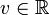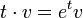# GAPlus(1,R)

View a complete list of particular groups (this is a very huge list!)[SHOW MORE]

## Definition

This group, denoted$GA^+(1,\R)$, is defined as the group (under composition) of functions$\R \to \R$ of the form:$x \mapsto ax + b, a,b \in \R, a > 0$

Equivalently, it can be defined as the external semidirect product$\R \rtimes (\R^*)^+$ where the latter is the multiplicative group of positive reals and it act on the former by multiplication.

Note that, via the logarithm map, the acting group is isomorphic to$\R$. Thus, the group can be defined as$\R \rtimes \R$ where$t \in \R$ (as an element of the acting group) acts on$v \in \R$ (as an element of the base group) by$t \cdot v = e^tv$.

The group is a subgroup of index two in general affine group:GA(1,R).

## Group properties

Property Satisfied? Explanation
abelian group No Follows from being centerless
nilpotent group No Follows from being centerless
centerless group Yes GAPlus(1,R) is centerless
metabelian group Yes
solvable group Yes
rationally powered group Yes GAPlus(1,R) is rationally powered